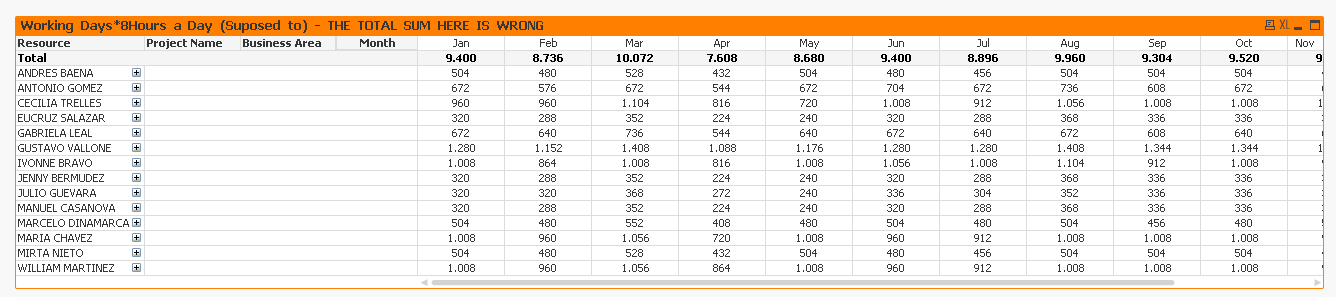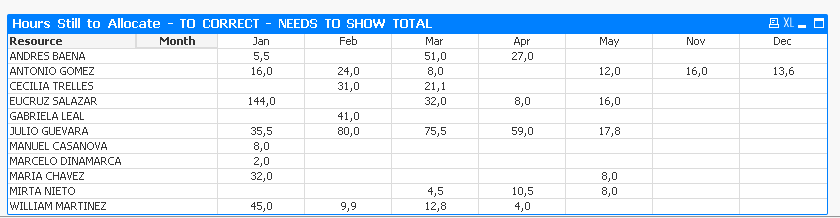# QlikView Creating Analytics

Discussion Board for collaboration related to Creating Analytics for QlikView.

Contributor

## Sum Total - Maybe with Aggregate

Hi All!

I have The total value within the lines are correct, but the total sum is wrong.

anyone know what change I need in the expression to have the right Total Sum?

My current expression is: Sum(DISTINCT(if(Month=Month_Working_Days,Working_Days_Qt*8)))Tags (1)
6 RepliesMVP

## Re: Sum Total - Maybe with Aggregate

May be this

Sum(Aggr(Sum(DISTINCT(if(Month=Month_Working_Days,Working_Days_Qt*8))), Resource, [Project Name], [Business Area], Month))HighlightedMVP

## Re: Sum Total - Maybe with Aggregate

Or this

If(Dimensionality() = 0, Sum(Aggr(Sum(DISTINCT(if(Month=Month_Working_Days,Working_Days_Qt*8))), Resource, Month)), Sum(DISTINCT(if(Month=Month_Working_Days,Working_Days_Qt*8))))Contributor

## Re: Sum Total - Maybe with Aggregate

Last one:

There is a conditional before and then the subtraction:

if(Sum([Hours Resource])/
Sum(DISTINCT(if(Month=Month_Working_Days,Working_Days_Qt*8)))
<=.99,

Sum(Aggr(Sum(DISTINCT(if(Month=Month_Working_Days,Working_Days_Qt*8)))
-
Sum([Hours Resource]),Resource,Month, [Resources Location])))Contributor

## Re: Sum Total - Maybe with Aggregate

What can I do to show the totals?MVP

## Re: Sum Total - Maybe with Aggregate

May be this

Sum(Aggr(if(Sum([Hours Resource])/

Sum(DISTINCT(if(Month=Month_Working_Days,Working_Days_Qt*8)))

<=.99,

Sum(Aggr(Sum(DISTINCT(if(Month=Month_Working_Days,Working_Days_Qt*8)))

-Sum([Hours Resource]),Resource,Month, [Resources Location]))),Resource,Month, [Resources Location]))

Contributor

## Re: Sum Total - Maybe with Aggregate

Totally worked! thanks a lot!